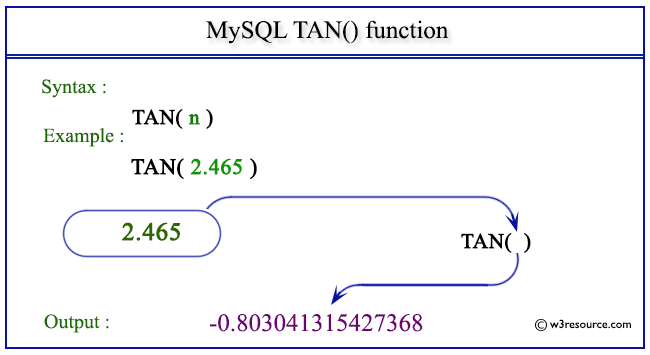# MySQL TAN() function

## TAN() function

MySQL TAN() returns the tangent of the argument. The argument is given in radians.

This function is useful in -

• TAN() is a fundamental trigonometric function that is used extensively in mathematics and engineering to solve problems related to angles, distances, and periodic phenomena.
• In geometry and physics, TAN() is crucial for calculating distances, angles, and trajectories.
• In computer graphics and animation, TAN() is used to generate smooth and realistic motion, especially in scenarios involving rotation and oscillation.
• TAN() is used in various simulations to model natural phenomena, such as the motion of pendulums, the behavior of springs, and the propagation of waves.
• TAN() might be used in algorithms that require understanding or manipulation of spatial relationships, which can be relevant in fields like computer vision and robotics.
• Engineers use TAN() for tasks like structural analysis, where understanding the behavior of materials under different loads and angles is essential.

Syntax:

```TAN(X);
```

Where X is a number

Syntax Diagram:MySQL Version: 8.0

Pictorial presentation of MySQL TAN() functionExample of MySQL TAN() function

Code:

``````SELECT TAN(2.465);
```
```

Explanation:

The above statement will return the tangent of the given number 2.465.

Output:

```mysql> SELECT TAN(2.465);
+--------------------+
| TAN(2.465)         |
+--------------------+
| -0.803041315427368 |
+--------------------+
1 row in set (0.00 sec)
```

All Mathematical Functions

Previous: SQRT()
Next: TRUNCATE()

﻿